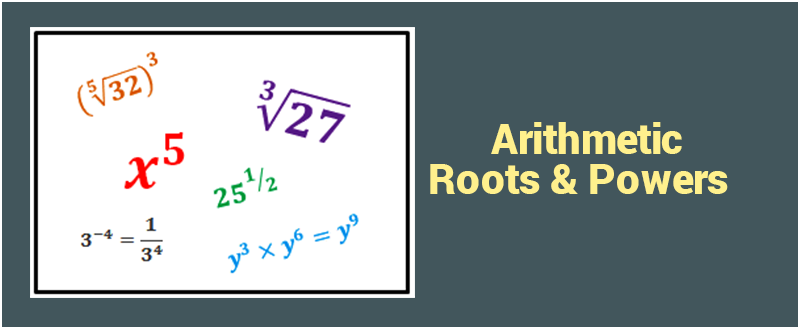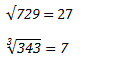# GMAT Quant: Arithmetic – Roots & PowersWe have been studying roots and powers since our grade school, still it never seizes to amaze us with the complexity of problem it presents.

## What are Powers?

Power is a way of indicating that a particular number is being multiplied by itself for some number of times. For example, if we express 36, then it is equivalent to 3  x 3 x 3 x 3 x 3 x 3 =  729.

## What are Roots?

Inverse of power is root.Read more GMAT Quant related articles.

Let’s solve a few questions and clear the basic concepts:

Example 1. If 22p+2 = 64, and, q = 2p+1 , Then find the value of q – p.

1. 3
2. 5
3. 10
4. 13
5. 17

Solution: 22p+2 = 26

So, 2p + 2 = 6

So, p = 2

Hence, q = 2p+1

q = 23 = 8

So, p + q = 2 + 8 = 10

Example 2 – $If \; \sqrt{3 – x} = \sqrt{x} + 3 \; Then, \; x^{2} = ?$

Solution: $(\sqrt{3 – x})^{2} = (\sqrt{x} + 3)^{2}$ $3 – x = x + 3 (\sqrt{x}) + 3 (\sqrt{x}) + 9$ $3 – x = x + 2 \times 3 (\sqrt{x}) + 9$ $3 – x = x + 6 (\sqrt{x}) + 9$ $2x + 6 \sqrt{x} + 6 = 0$ $2 (x + 3 \sqrt x + 3)$ It can be written as,
$x + 3 \sqrt x + 3 = 0$ $- 3 \sqrt x = x + 3$ $(- 3 \sqrt x)^{2} = (x + 3)^{2}$ $9x = x^{2} + 6x + 9$ $x^{2} -3x + 9 = 0$ Therefore, $x^{2} = -3x + 9$

Want to score 800 in GMAT? Click to read more. You can also call us @ +918884544444 or write to us. BYJU’s mentors will be more than happy to guide you to crack this Giant GMAT.